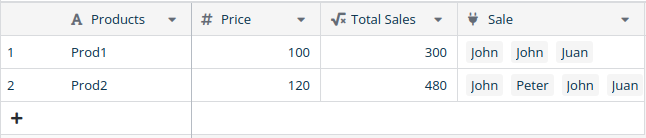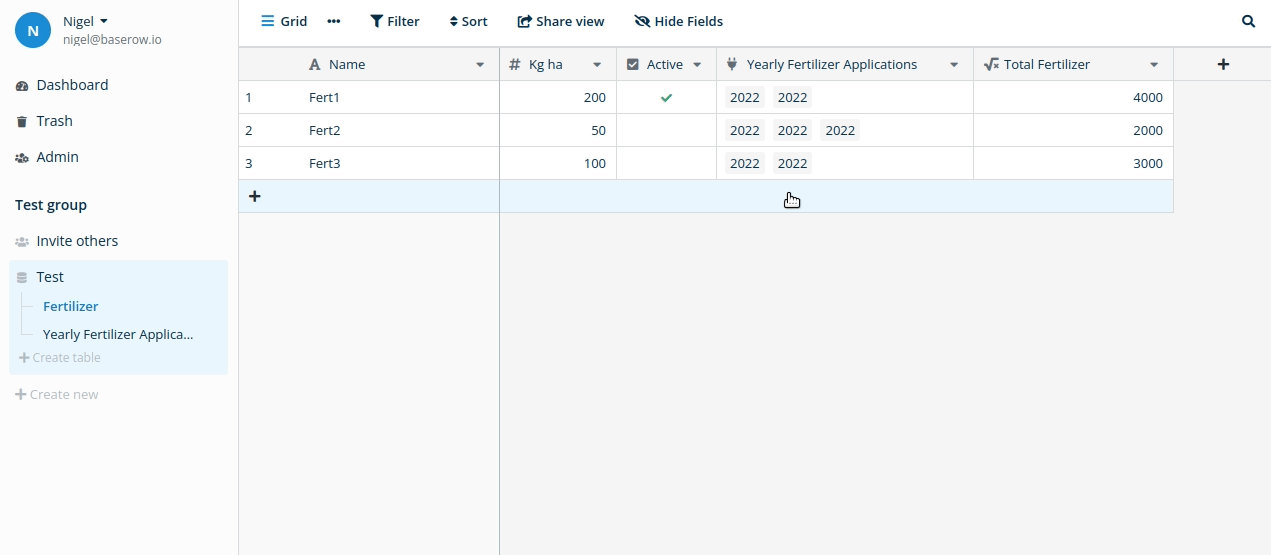Sum(lookup) from another table with more than one entry per field

First of all, I congratulate the Baserow developers for the ease of use and speed that an application can be made.
How can I add the total sales per product in the product table from the sales table, where I have more than one product per field and also more than one price per fieldHey @ast ,

Not 100% sure what you mean, in your example above are you asking what the formula should be for the Total Sales column? If so then here I would imagine it would be count(field('Sale'))*field('Price') . If not could you also show a picture of the Sale table and it’s fields and point out which exact field you are looking to figure out the formula for with some expected results?

Thanks,
Nigel

The result that appears in the Products table “Total Sales” I obtained it with count (field (‘Sale’)) * field (‘Price’), as you say, but what I want is to obtain the same result but from the table Sale

So in the second table you screenshotted you would like to have a Sale Total column where it is the total value of that specific sale? And would look like:

+-------+--------+------------+
| RowId |  Name  | Sale Total |
+-------+--------+------------+
|     1 | John   |        220 |
|    3  | Peter  |        120 |
|    4  | John   |        220 |
|    5  | Juan   |        220 |

If so the formula would be sum(field('Price')) .

Or perhaps you want to see in the Sale table a field with for every product in that sale the sum of all the sales for that product? Then I guess you could make a formula field in the Sale table with formula sum(lookup('Prod', 'Total Sales')).

1 Like

First of all, thank you for your prompt response, in order to better explain what I need I will explain you in more detail.

How can I obtain with a formula the “Total Fertilizer” in the “Fertilizer” Table something like sum (lookup (“Apply”, “Total Fertilizer”))
Thanks

Ah I see what you mean now, I think this does what you want:

sum(lookup('Yearly Fertilizer Applications', 'Hectares apply')*field('Kg ha'))1 Like

Perfect thanks, have a nice day